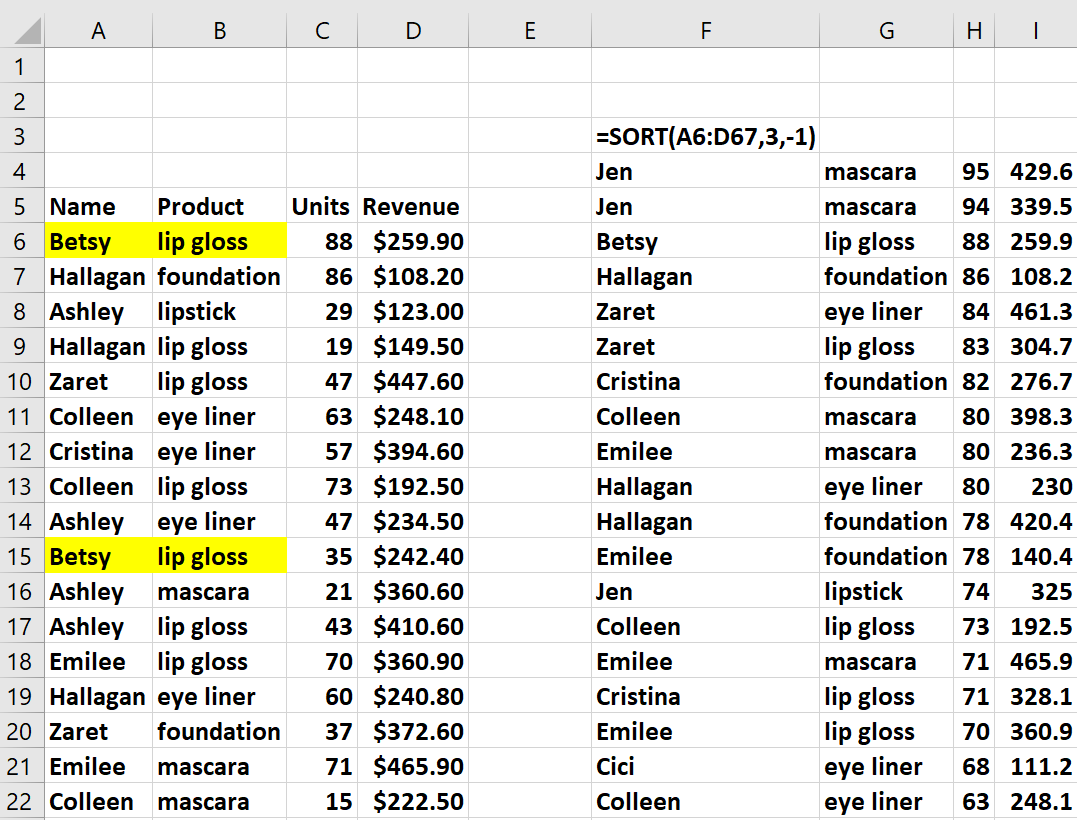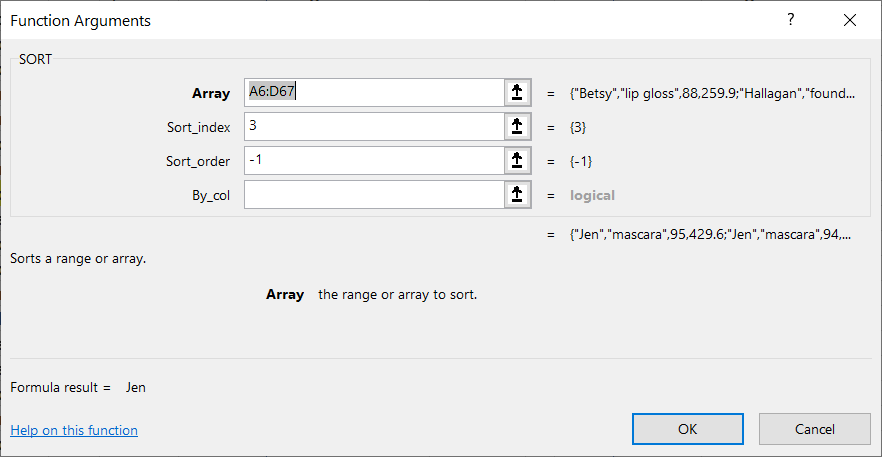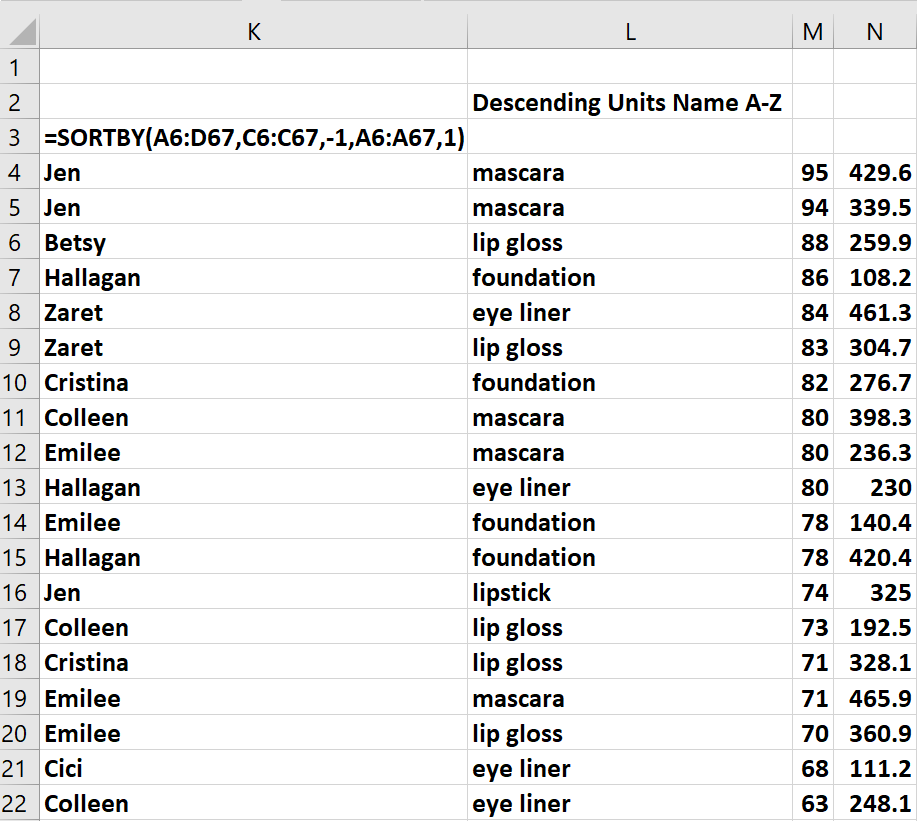# The Amazing SORT and SORTBY FunctionsIf you have Office 365 (recently renamed as Microsoft 365), you now have access to several amazing dynamic array functions that will enable you to perform many helpful tasks. In this article we will give you several examples that illustrate the power of the SORT and SORTBY dynamic array functions.

Usually we sort using DATA SORT/ or the A to Z /  Z to A button. However, the SORT and SORTBY functions enable you to sort data using a function. If your source data is an Excel table, then these formulas automatically update if new data is added.

This Excel workbook contains several examples of these amazing functions.

In the worksheet “Original” (see Figure 1), the range A5:D67 contains 4 pieces of information for 63 sales transactions:

• Salesperson name

• Product Sold

• Units Sold

• Revenue from Transaction

Figure 1 - Example of Sort FunctionThe formula =SORT(A6:D67,3-1) returns data sorted in descending order from 3rd column (Units). This dynamic array formula knows to “fill down” all the data in the correct order! Thus F4:I4, the first row, gives the data for the transaction involving the most units, while F65:I65, the last row, gives the data for the transaction involving the fewest units. Figure 2 shows the syntax for the formula.

Figure 2  - Syntax of SORT function• The array A6:D67 contains the data to be sorted.
• The Sort_index of 3 indicates the sort is based on the 3rd column of data.
• Sort_Order of -1 indicates sort in descending order. A Sort Order of +1 or omitted indicates sort in ascending order.
• Omitting the By_col argument  or entering FALSE creates a sort by row. An argument of TRUE creates a sort across columns.

As shown in Figure 3, the formula entered in cell K3, =SORTBY(A6:D67,C6:C67,-1,A6:A67,1) sorts our data in descending order by units and then by Name A-Z. Note that rows 11-13 all have 80 units sold, so they are listed based on an A-Z sort of the salesperson’s name.

Figure 3 - Sort by Units (Descending) and Names A-ZNote that the first SortOrder argument of -1 results in a sort of units (based on C6:C67) in descending order while the second Sort_Order  argument of +1 results in ties in unit sales being broken by an A-Z sort based on the salesperson.

Next month we will introduce you to the amazing FILTER function which enables you to use a formula (instead of the Excel “filter” icon) to filter an Excel database.